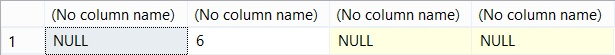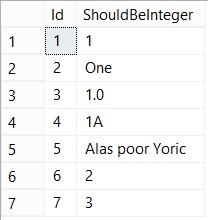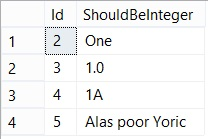TRY_CONVERT

,

For the entire history of T-SQL, we’ve had the built-in CONVERT function to convert values from one data type to another. This is great, but sometimes we don’t know ahead of time whether the value we want to convert will be valid in our target data type. This could happen, for instance, where we are manipulating data from a source we don’t have control over, and where invalid values or formats of data sometimes slip through.

Where that happens with CONVERT, an error would be generated. We can try and avoid that by carefully validating the data before we attempt the conversion, but that can get complicated. In SQL Server 2012 and later versions, we have an easier option. TRY_CONVERT performs exactly the same as CONVERT and has the same syntax, except that if the conversion fails then it doesn’t raise an error, instead a Null value is returned.

A lot of the time this is exactly what we want, convert the value if you can, but if you can’t then let me deal with that separately. Certainly, where I am converting a bunch of rows I often want the ones that are valid to succeed, rather than an error being raised that rolls back the whole update.

Note though that TRY_CONVERT will return an error if you try an invalid conversion, i.e. from one data type to another that are not compatible – such as from an integer value to XML.

Let’s look at some quick examples of the difficulties we might encounter using the CONVERT function, and then we’ll see how that is fixed with TRY CONVERT.

`SELECT CONVERT(INT, 'Not a Number');`

When I execute this, I get the following error:

`Msg 245, Level 16, State 1, Line 1 `

`Conversion failed when converting the varchar value 'Not a Number' to data type int. `

Now I could try and validate my string value before the conversion using the ISNUMERIC function. We’ll put the value we want to convert into a variable to make this more re-usable:

```DECLARE @SomeText VARCHAR(50) = 'Not A Number';
IF ISNUMERIC(@SomeText) = 1
BEGIN
SELECT CONVERT(INT, @SomeText);
END
ELSE BEGIN
SELECT NULL;
END```

This returns Null, so that is great. If I change the value of @SomeText to a valid integer value e.g. ‘6’, then the query returns that value. What if I try passing a decimal though e.g. ‘1.23’:

`Msg 245, Level 16, State 1, Line 17 `

`Conversion failed when converting the varchar value '1.23' to data type int. `

Hmmm, looks like I need more validation to make sure we have an integer, not a decimal. Worse, ISNUMERIC will permit characters that could appear in numeric types such as commas, full stops and currency symbols e.g.

`SELECT ISNUMERIC(',');`

Returns the value 1 – but our conversion will still fail:

`Msg 245, Level 16, State 1, Line 17 `

`Conversion failed when converting the varchar value ',' to data type int. `

With TRY_CONVERT, it’s simple. Let’s look at what we get if we try converting each of the values mentioned above:

```SELECT
TRY_CONVERT(INT, 'Not a Number'),
TRY_CONVERT(INT, '6'),
TRY_CONVERT(INT, '1.23'),
TRY_CONVERT(INT, ',');```

Gives us the results:You can see that only the valid integer value has been converted, and for the rest of the cases, a Null has been returned.

This isn’t just useful where we want to convert values that are valid and ignore the others, we can also use this to identify problem rows\values before we run an update. Let’s say we have the following table dbo.UncleanData that has some troublesome data:I can easily identify the rows that don’t contain valid integers:

```SELECT Id, ShouldBeInteger
FROM dbo.UncleanData
WHERE TRY_CONVERT(INT, ShouldBeInteger) IS NULL;```

And I get the results:It’s worth noting that TRY_CONVERT doesn’t only catch errors where the value being converted is not valid in the target type, e.g. converting text to a number, but also where the value would generate another error such as an overflow.

For instance:

`SELECT CONVERT(TINYINT, '999');`

Gives us the error:

`Msg 244, Level 16, State 1, Line 69 `

`The conversion of the varchar value '999' overflowed an INT1 column. Use a larger integer column. `

Whereas:

`SELECT TRY_CONVERT(TINYINT, '999');`

Returns a Null value.

Of course, like CONVERT, TRY_CONVERT isn’t limited just to converting values to integers, you can convert to other numeric types, dates etc. Let’s look at some more examples:

First, using decimals instead of integers:

```SELECT TRY_CONVERT(DECIMAL(5,2), '12.345');  --Returns 12.34
SELECT TRY_CONVERT(DECIMAL(5,2), '123.45');  --Returns 123.45
SELECT TRY_CONVERT(DECIMAL(5,2), '123.456'); --Returns 123.46
SELECT TRY_CONVERT(DECIMAL(5,2), '999.99');  --Returns 999.99
SELECT TRY_CONVERT(DECIMAL(5,2), '1000.00'); --Returns Null
SELECT TRY_CONVERT(DECIMAL(5,2), '1234.5');  --Returns Null
SELECT TRY_CONVERT(DECIMAL(5,2), '12345');   --Returns Null
SELECT TRY_CONVERT(DECIMAL(5,2), 'Fred');    --Returns Null```

Just remember when you're converting decimals (using the DECIMAL or NUMERIC types) that you may be converting a valid value, but your target type must be large enough to store it.

In the above examples I've used a DECIMAL(5,2), that allows for 5 digits in total (the precision) and 2 digits after the decimal point (the scale). As such, there are only 3 digits available before the decimal point so any conversion with more that that will fail.

As you can see above, 999.99 was successful, but after that the numeric values were too big and so a Null value was returned.

Here’s some more examples, involving dates this time:

```SELECT TRY_CONVERT(DATE, '12:53:00.000');          --Returns 1900-01-01
SELECT TRY_CONVERT(DATE, '20180201');              --Returns 2018-02-01
SELECT TRY_CONVERT(DATE, '20180228 12:53:00.000'); --Returns 2018-02-28
SELECT TRY_CONVERT(DATE, '20180229');   --Returns Null (As 2018 wasn't a leap year)
SELECT TRY_CONVERT(DATE, 'Not a Date'); --Returns Null```

Conclusion

TRY_CONVERT is a simple way of performing conversions from one data type to another while avoiding errors, or for easily identifying rows in a table that will fail to convert if you make the attempt.

It’s worth noting that we also have the TRY_CAST function, which is to the CAST function what TRY_CONVERT is to CONVERT.

As mentioned, TRY_CONVERT will generate an error if you attempt to convert between incompatible data types. You can see which conversions are valid, as well as find reference for the optional style parameter in books online:

4.93 (27)

4.93 (27)